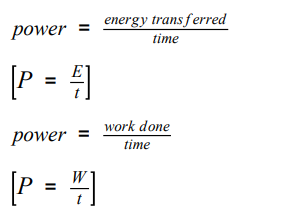# AQA GCSE Physics Power focus questions

## Focus questions on power for AQA GCSE Physics

1. Copy and fill in the blanks. Power is defined as the __________ at which energy is ___________or the rate at which _______ is done.
1. Consider these equations.2. Can you see by comparing the equations that work done = energy transferred?
3. What is the unit of power?
4. What is the unit of energy?
5. What is the unit of work done?
6. What is the unit of time?
7. Rearrange the equations so that. E =, t =
8. Rearrange the equations so that W = and t =
9. Copy the following: An energy transfer of 1 joule per second is equal to a power of 1 watt.